C Program to Multiply Two Matrices

In this tutorial you will learn about the C Program to Multiply Two Matrices and its application with practical example.

C Program to Print Multiply Two Matrices

In this tutorial, we will learn to create a C program that will Print Multiply Two Matrices  C programming.

Prerequisites

Before starting with the tutorial we assume that you are best aware of the following C programming topics:

• Operators in C Programming.
• Basic Input and Output function in C Programming.
• Basic C programming.
• For loop in C programming.

Program to Print Multiply Two Matrices

In c programming, it is possible to take a numerical input for the size of the matrix and the elements of the matrix from the user and Print Multiply Two Matrices with the help of a very small amount of code. The C language has many types of header libraries which has supported function in them with the help of these files the programming is easy.

Algorithm:-

With the help of this program, we can Print Multiply Two Matrices.

Output:-In the above program, we have first initialized the required variable.

• a= it will hold the integer value.
• b= it will hold the integer value.
• mul= it will hold the integer value.
• r= it will hold the integer value.
• c= it will hold the integer value.
• k= it will hold the integer value.
• i= it will hold the integer value.
• j= it will hold the integer value.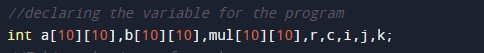Taking the size of the matrix from the user.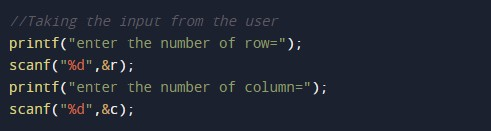Input elements of both the matrices.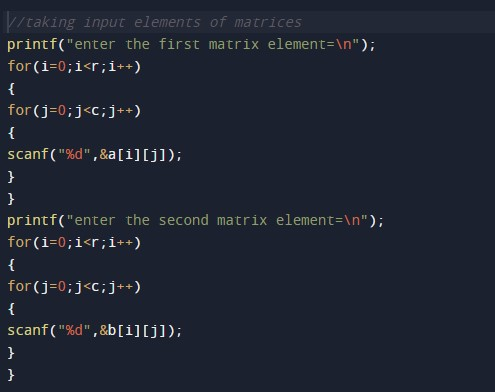Calculating the multiply of the two matrices.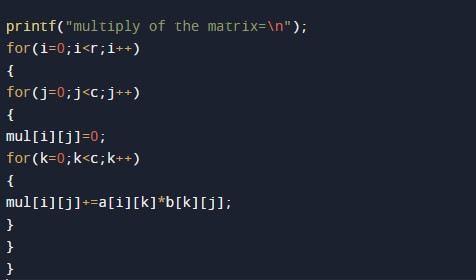Printing the output for the program.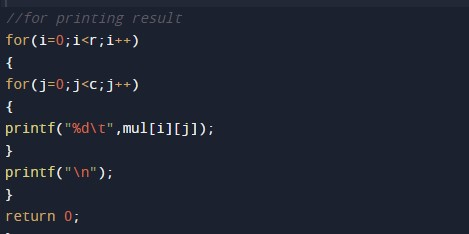In this tutorial we have learn about the C Program to Multiply Two Matrices and its application with practical example. I hope you will like this tutorial.